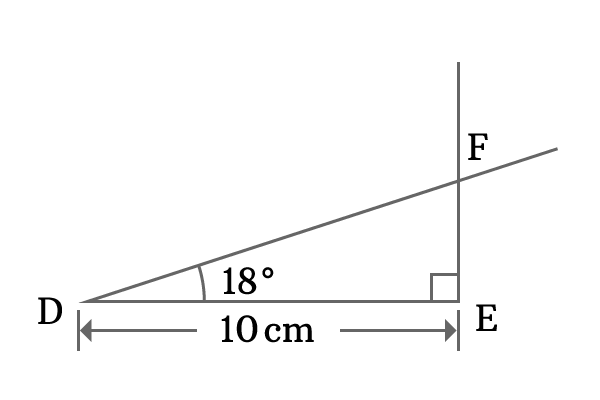# cos 18°

$\cos 18^\circ$ $=$ $\dfrac{\sqrt{10 + 2\sqrt{5}}}{4}$ $=$ $0.951056516\ldots$

## Proof

Theoretically, it is not possible to find the value of cos of $18$ degrees directly. Alternatively, it can be found from the value of sine $18$ degrees. Therefore, use $\sin 18^\circ$ value and transform that value into $\cos 18^\circ$ by the trigonometric relation between sine and cosine functions.

Use Pythagorean identity between sine and cosine and express cosine in terms of sine.

$\cos \theta = \sqrt{1 -\sin^2 \theta}$

Here, the angle theta is $18^\circ$.

$\implies \cos 18^\circ = \sqrt{1 -\sin^2 18^\circ}$

Now replace sin 18 degrees by its value in the equation.

$\implies \cos 18^\circ$ $=$ $\sqrt{1 -{\Bigg(\dfrac{\sqrt{5}-1}{4}\Bigg)}^2}$

$\implies \cos 18^\circ$ $=$ $\sqrt{1 -\dfrac{{(\sqrt{5}-1)}^2}{4^2} }$

$\implies \cos 18^\circ$ $=$ $\sqrt{1 -\dfrac{{(\sqrt{5})}^2 + {(1)}^2 -2 \times \sqrt{5} \times 1}{16}}$

$\implies \cos 18^\circ$ $=$ $\sqrt{1 -\dfrac{5 + 1 -2\sqrt{5}}{16}}$

$\implies \cos 18^\circ$ $=$ $\sqrt{1 -\dfrac{6 -2\sqrt{5}}{16}}$

$\implies \cos 18^\circ$ $=$ $\sqrt{\dfrac{16 -(6 -2\sqrt{5})}{16}}$

$\implies \cos 18^\circ$ $=$ $\sqrt{\dfrac{16 -6 + 2\sqrt{5}}{16}}$

$\implies \cos 18^\circ$ $=$ $\sqrt{\dfrac{10 + 2\sqrt{5}}{16}}$

$\implies \cos 18^\circ = \dfrac{\sqrt{10 + 2\sqrt{5}}}{4}$

$\therefore \,\,\,\,\,\,\, \cos 18^\circ$ $=$ $\dfrac{\sqrt{10 + 2\sqrt{5}}}{4}$ $=$ $0.951056516\ldots$

### Verification

The value of cos of angle $18$ degrees can also be derived by constructing a right angled triangle with angle $18^\circ$.1. Draw a horizontal line of any length by using ruler. For example, $10$ cm line is drawn and it is called line $\overline{DE}$.
2. Use protractor and draw a perpendicular line to line segment $\overline{DE}$ at point $E$.
3. Use protractor and draw a line from point $D$ with an angle of $18$ degrees and the $18^\circ$ line intersects the perpendicular line at point $F$.

Thus, a right angled triangle, known as $\Delta FDE$ is constructed geometrically. The angle of the right angled triangle is $18^\circ$. So, find the value of $\cos 18^\circ$.

$\cos 18^\circ = \dfrac{DE}{DF}$

Geometrically, the length of the adjacent side is $DE$ and it is $10$ cm but the length of the hypotenuse $DF$ is unknown. However, it can be measured by using ruler. It is measured that the length of the hypotenuse is $10.5$ cm.

$\cos 18^\circ = \dfrac{10}{10.5}$

$\cos 18^\circ = 0.952380952\cdots$

#### Conclusion

According to theoretical approach, the value of $\cos 18^\circ$ is $0.951056516\ldots$ and the value of $\cos 18^\circ$ is $0.952380952\cdots$ as per geometrical method.

The two values of cos of angle $18$ degrees from these two methods are approximately same but the value of $\cos 18^\circ$, obtained from theoretical approach is true value and the value of cos $18$ degrees, obtained from geometrical method cannot be considered as true value.

In geometric method, the length of the adjacent side is exact value but the length of the hypotenuse is an approximate length and it affects the ratio of them. Thus, the value of $\cos 18^\circ$ in geometric method is an approximate value.

Therefore, it is verified that the value of $\cos 18^\circ$ is $\dfrac{\sqrt{10 + 2\sqrt{5}}}{4}$ in surd form and $0.951056516\ldots$ in decimal form.

Latest Math Topics
Latest Math Problems
Email subscription
Math Doubts is a best place to learn mathematics and from basics to advanced scientific level for students, teachers and researchers. Know more
Follow us on Social Media
###### Math Problems

Learn how to solve easy to difficult mathematics problems of all topics in various methods with step by step process and also maths questions for practising.

Learn more